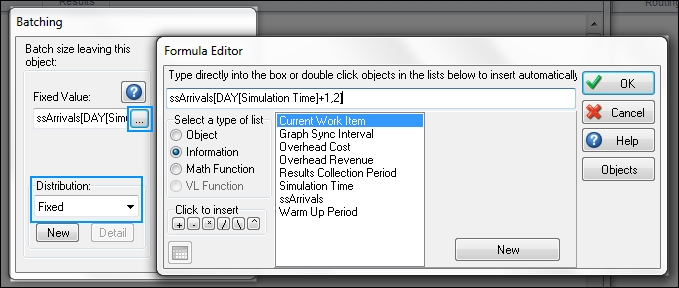Written by on Friday August 30th 2013 in SIMUL8 Tips, Technical Corner

## SIMUL8 Tip – Use a Spreadsheet to Generate Batched Arrivals by Day

Many users found using a CSV to generate arrivals very useful, so we decided we’d write a similar tip but instead using internal spreadsheets as an interface. This can be used in scenarios where large quantities of goods come at a regular interval, and where the quantities remain static.

The first thing we will do is create an internal spreadsheet called ssArrivals. To do this, navigate to the Data and Rules Tab, and then select Information Store.We now want to set up our spreadsheet like in the image below. This is to create an interface that other users can then use to input new data to test different scenarios of arrival amounts.Once the spreadsheet is set up, we now need to tell the Start Point to read these values from the spreadsheet. To do this, select the Start Point and then navigate to the Properties Tab and select Batching Out. We are going to create a formula to reference the spreadsheet, and we will want to set the distribution to Fixed.The formula to use is as follows:

ssArrivals[DAY[Simulation Time]+1,2]

Let’s break down this formula a bit:

ssArrivals is the name of the spreadsheet we want to read from.

For the column, we have written DAY[Simulation Time]+1. DAY is a math function within SIMUL8 that will converts the number from time to day 1-7 calculated from the Clock Properties. That means, with each new day in our simulation, SIMUL8 will automatically move its reference to the next column.

The +1 part of this formula is to offset the column, as the 1st column does not contain any relevant information, so we want to start on the 2nd column.
Finally, the 2 represents the row we are reading from, and in this example we will always be reading form the 2nd row of our spreadsheet.

You will then need to ensure that the “First At Start” option is enabled at the Start Point. Select the Start Point, navigate to the Additional Tab, and then check First At Start.

Finally, we need to set the timing for the Start Point. Use a Fixed distribution with an interarrival rate of the number of hours in your day. For example, if you have an 8 hour day and your simulation runs in hours, then your interarrival rate is simply 8. If you have an 8 hour day but run your simulation in minutes, then you can use 480 as your rate (8 hours * 60 minutes).

We hope this helps provide a useful way to generate your arrivals. If you have any questions, then please leave us a comment below!